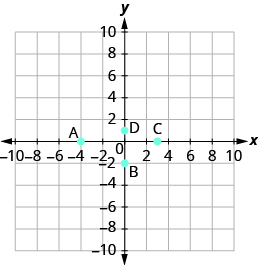## What you’ll learn to do: Plot lines on the rectangular coordinate system using x- and y-intercepts

One of the easiest ways to graph a line is by using the x- and y- intercepts as two of your points.

In this section, we will learn how to:

• Identify the x- and y-intercepts on a graph and as ordered pairs
• Find the intercepts from an equation of a line
• Graph a linear equation using x and y-intercepts

Before you get started in this module, try a few practice problems and review prior concepts.

1)

Solve: $3x+4y=-12$ for $x$ when $y=0$.

Solution: $x=-4$

2)

If you missed this problem, review this example.

Name the ordered pair of each point shown:3)

If you missed this problem, review the example below.

Determine which ordered pairs are solutions of the equation $x+4y=8\text{:}$

1. $\left(0,2\right)$
2. $\left(2,-4\right)$
3. $\left(-4,3\right)$

## Contribute!

Did you have an idea for improving this content? We’d love your input.